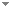Switch to: References

# Two Decision Procedures for da Costa’s $$C_n$$ C n Logics Based on Restricted Nmatrix Semantics

Studia Logica 110 (3):601-642 (2022)

 Two Decision Procedures for da Costa’s $$C_n$$ C N Logics Based on Restricted Nmatrix Semantics.Marcelo E. Coniglio & Guilherme V. Toledo - 2022 - Studia Logica 110 (3):601-642.Despite being fairly powerful, finite non-deterministic matrices are unable to characterize some logics of formal inconsistency, such as those found between mbCcl and Cila. In order to overcome this limitation, we propose here restricted non-deterministic matrices (in short, RNmatrices), which are non-deterministic algebras together with a subset of the set of valuations. This allows us to characterize not only mbCcl and Cila (which is equivalent, up to language, to da Costa's logic C_1) but the whole hierarchy of da Costa's calculi (...) Direct download (4 more)     Export citationBookmark3 citations Weakly Free Multialgebras.Marcelo Esteban Coniglio & Guilherme Vicentin de Toledo - 2022 - Bulletin of the Section of Logic 51 (1):109-141.In abstract algebraic logic, many systems, such as those paraconsistent logics taking inspiration from da Costa's hierarchy, are not algebraizable by even the broadest standard methodologies, as that of Blok and Pigozzi. However, these logics can be semantically characterized by means of non-deterministic algebraic structures such as Nmatrices, RNmatrices and swap structures. These structures are based on multialgebras, which generalize algebras by allowing the result of an operation to assume a non-empty set of values. This leads to an interest in (...)No categories Direct download (2 more)     Export citationBookmarkIn a previous article we introduced the concept of restricted Nmatrices (in short, RNmatrices), which generalize non-deterministic (in short, Nmatrices) in the following sense: a RNmatrix is a Nmatrix together with a subset of valuations over it, from which the consequence relation is defined. Within this semantical framework we have characterized each paraconsistent logic Cn in the hierarchy of da Costa by means of a (n+2)-valued RNmatrix, which also provides a relatively simple decision procedure for each calculus (recalling that C1 (...) Direct download     Export citationBookmark